# Will I ever be a bayesian statistician ? (part 1)

January 20, 2011
By

Want to share your content on R-bloggers? click here if you have a blog, or here if you don't.

Last week, during the workshop on Statistical Methods for
Meteorology and
Climate Change
(here),
I discovered how powerful bayesian techniques could be, and that there
were more and more bayesian statisticians. So, if I was to fully
understand
applied statisticians in conferences and workshops, I really have to
understand basics of bayesian statistics. I have published some time
ago some posts on bayesian statistics applied to actuarial problems (here or there), but so far, I always thought that bayesian was a synonym for magician.
To be honest, I am a Muggle, and I have not been trained as a bayesian. But I can be an opportunist…
So I decided to publish some posts on bayesian techniques, in order to
prove that it is actually not that difficult to implement.As far as I understand it, in bayesian statistics, the parameter
is considered as a random variable (which is also the case, in classical mathematical statistics). But here, here assume that this parameter does have a
parametric distribution….
Consider a classical statistical problem: assume we have a samplei.i.d. with distribution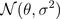. Here we notesince parameterthe is a random variable. The idea is to assume thathas a (so called priori)
distribution, e.g.So far it was simple. The idea is then to consider the posterior distribution of, given the observations. Thus, we need to compute the distribution ofwhich is here extremely simple (due to properties of the Gaussian distribution), i.e.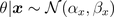whereAnd them, it becomes extremely natural to consideras an estimator of given our sample data (and thus, we also have a confidence interval since we know the distribution ofgiven the observations).
In order to be sure that we understood, consider now a heads and tails problem, i.e.. Note, first, that theta has support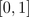. So we need a distribution
on that support. Why not a beta distribution ? E.g.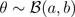Thus,andFrom Bayes formula,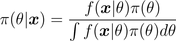and we get easily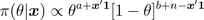which is the density of a Beta distribution, i.e.`prior=dbeta(u,a,b)posterior=dbeta(u,a+y,n-y+b)`

The estimator proposed is then the expected value of that conditional
distribution,Note thatFurther, it is possible to derive confidence intervals using quantiles
of the posterior distribution.
On the graphs below, we consider the following heads/tails sample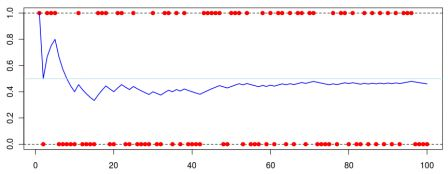A first idea is to consider a uniform prior distribution.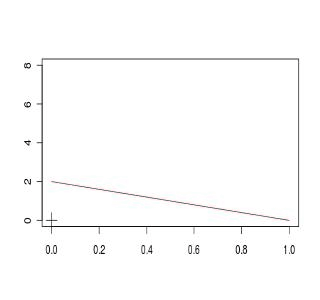A second idea is to consider an asymmetric beta distribution. First, with an asymmetry on the left,or on the right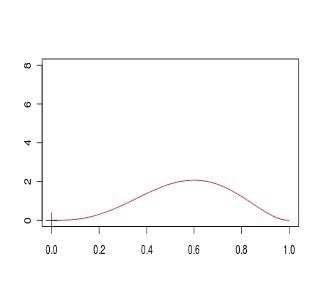Finally a third idea is simply to get back to the standard Gaussian
approximation,If we compare the four models, we obtain (the plain black line is the Gaussian approximated distribution for the empirical mean), and red lines are obtained from prior beta distributionsThe code to generate those graphs is the following
`a1=1; b1=1D1[1,]=dbeta(u,a,b)a2=4; b2=2D2[1,]=dbeta(u,a,b)a3=2; b3=4D3[1,]=dbeta(u,a,b)setseed(1)S=sample(0:1,size=100,replace=TRUE)COULEUR=rev(rainbow(120))D1=D2=D3=D4=matrix(NA,101,length(u))for(s in 1:100){y=sum(S[1:s])D1[s+1,]=dbeta(u,a1+y,s-y+b1)D2[s+1,]=dbeta(u,a2+y,s-y+b2)D3[s+1,]=dbeta(u,a3+y,s-y+b3)D4[s+1,]=dnorm(u,y/s,sqrt(y/s*(1-y/s)/s))plot(u,D1[1,],col="black",type="l",ylim=c(0,8),xlab="",ylab="")for(i in 1:s){lines(u,D1[1+i,],col=COULEUR[i])}points(y/s,0,pch=3,cex=2)plot(u,D2[1,],col="black",type="l",ylim=c(0,8),xlab="",ylab="")for(i in 1:s){lines(u,D2[1+i,],col=COULEUR[i])}points(y/s,0,pch=3,cex=2)plot(u,D3[1,],col="black",type="l",ylim=c(0,8),xlab="",ylab="")for(i in 1:s){lines(u,D3[1+i,],col=COULEUR[i])}points(y/s,0,pch=3,cex=2)plot(u,D4[1,],col="white",type="l",ylim=c(0,8),xlab="",ylab="")for(i in 1:s){lines(u,D4[1+i,],col=COULEUR[i])}points(y/s,0,pch=3,cex=2)plot(u,D4[s+1,],col="black",lwd=2,type="l",ylim=c(0,8),xlab="",ylab="")lines(u,D1[1+i,],col="blue")lines(u,D2[1+i,],col="red")lines(u,D3[1+i,],col="purple")points(y/s,0,pch=3,cex=2)}`

Here, we can see that computations are simple if the prior distribution
has a distribution which is the conjugate of the observations’
distribution (see here for the list of prior and posterior standard distributions).
So far, I have two questions that naturally show up

• is it possible to start with a neutral prior distribution, non informative ?
• what if we are no longer working with conjugate distributions ?

Well, I guess I have to work a bit more to answer those questions…. to be continuedR-bloggers.com offers daily e-mail updates about R news and tutorials about learning R and many other topics. Click here if you're looking to post or find an R/data-science job.
Want to share your content on R-bloggers? click here if you have a blog, or here if you don't.

Tags: , , , , , , , , ,